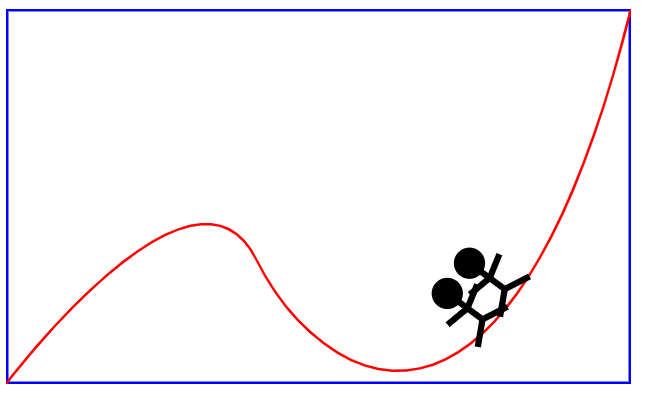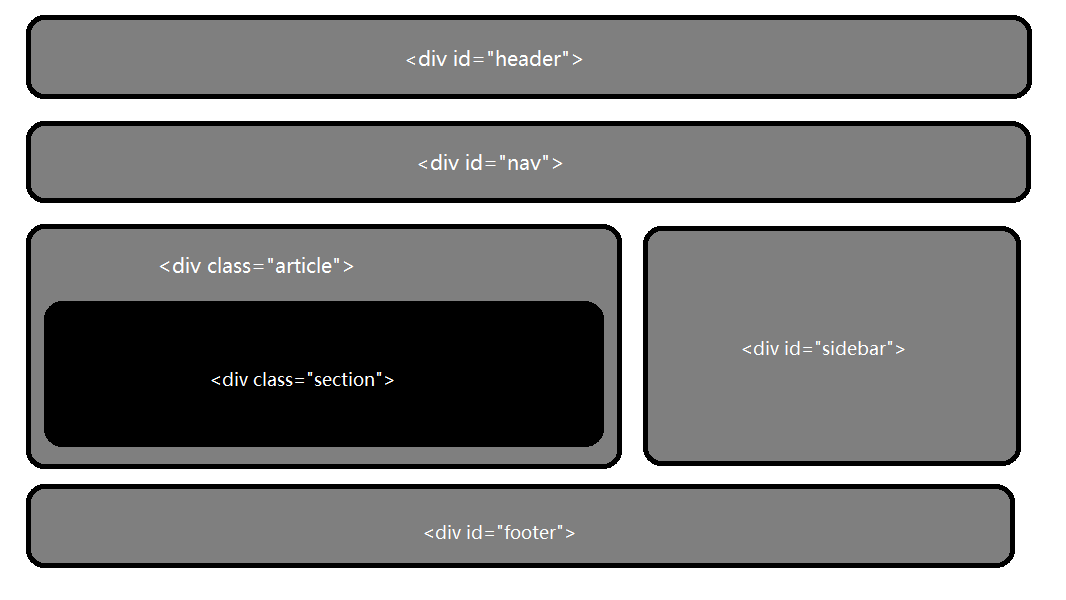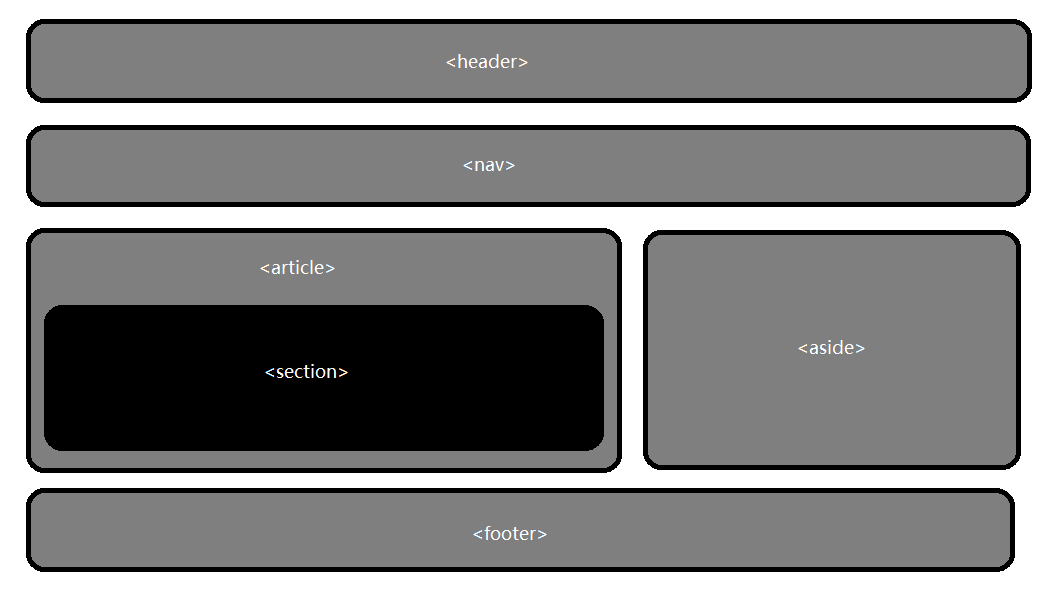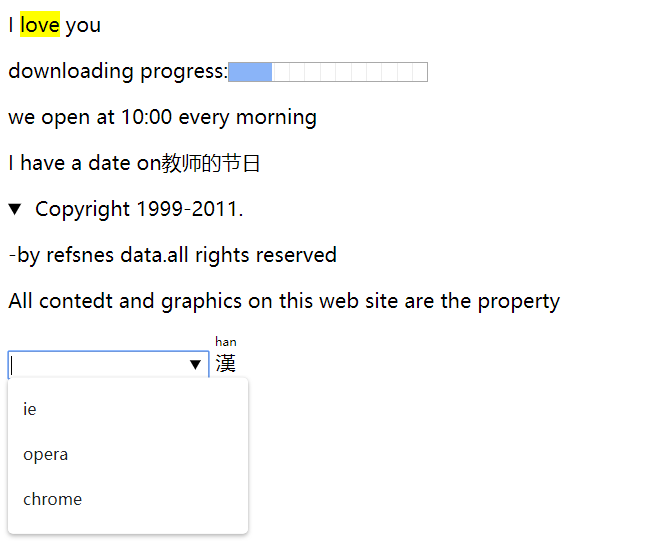# HTML5部分基礎知識

web前端開發 一個XML的簡單應用 代碼如下： 1 <?xml version="1.0" encoding="utf-8"?> 2 3 <svg width="500" height="300" xmlns="http://www.w3.org/2000/svg"> 4 <! > 5 <rect ...

web前端開發

代碼如下：

``` 1 <?xml version="1.0" encoding="utf-8"?>
2
3 <svg width="500" height="300" xmlns="http://www.w3.org/2000/svg">
4 <!---->
5     <rect x="1" y="1" width="498" height="298" fill="none" stroke="blue" stroke-width="2"/>
6 <!--    -->
7     <path d="M0,300 S150,100 200,200 S400,400 500,0" fill="none" stroke="red" stroke-width="2"/>
8 <!---->
9     <g stroke-width="5" stroke="black">
10 <!--    -->
11         <circle cx="0" cy="-45" r="10" fill="black"/>
12         <line x1="-20" y1="-30" x2="0" y2="-25"/>
13         <line x1="20" y1="-30" x2="0" y2="-25"/>
14         <line x1="-20" y1="0" x2="0" y2="-10"/>
15         <line x1="20" y1="0" x2="0" y2="-10"/>
16         <line x1="0" y1="-10" x2="0" y2="-45"/>
17
18     <circle cx="-30" cy="-45" r="10" fill="black"/>
19         <line x1="-50" y1="-30" x2="-30" y2="-25"/>
20         <line x1="-10" y1="-30" x2="-30" y2="-25"/>
21         <line x1="-50" y1="0" x2="-30" y2="-10"/>
22         <line x1="-10" y1="0" x2="-30" y2="-10"/>
23         <line x1="-30" y1="-10" x2="-30" y2="-45"/>
24 <!---->
25         <animateMotion path="M0,300 S150,100 200,200 S400,400 500,0" dur="8s" repeatCount="indefinite" rotate="auto"/>
26 </g>
27
28 </svg>```HTML5新增常用結構性元素

•  新增的結構性元素

section：定義文檔中的節。比如章節、頁眉、頁腳或文檔中的其他部分。一般用於成節的內容，會在文檔流中開始一個新的節。

article：它是一個特殊的section標簽，它比section具有更明確的語義，它代表一個獨立的、完整的相關內容塊，可獨立於頁面其他內容使用。

nav：此標簽代表頁面的一個部分，表示頁面中導航鏈接的部分

aside：它用來裝載非正文的內容，被視為頁面裡面一個單獨的部分。它包含的內容與頁面的主要內容事分開的，可以被刪除，而不會影響到網頁的內容、章節或是頁面所要傳達的信息。

hgroup：hgroup標簽是堆網頁或區段section的標題元素（h1-h6）進行組合。

footer：它定義section或文檔的頁腳，包含了頁面、文章或是部分內容有關的信息。

figure：用於對元素進行組合。多用於圖片與圖片描述組合。

• 在之前的HTML頁面中，大家基本上都是用了Div+CSS定義的佈局方式•  在之前的HTML頁面中，大家基本上都是用了Div+CSS佈局方式，而這個則精簡了許多•  新增的常用語義性元素

mark：定義帶有標記的文本，需要突出顯示文本時使用<mark>標簽。

progress：標簽定義運行中的任務進度（進程）.

time：表示時間日期值。

datalis：此標簽規定了用戶可見的或隱藏的需求的補充細節。

datalist：定義選項列表。請與input元素配合使用你該元素，來定義input可能的值。

ruby：此標簽定義ruby註釋，往往與<rt>和<rp>標簽一起配合使用。

command：commend標簽可以定義用戶可能調用的命令（比如單選按鈕、覆選按鈕或按鈕）。

2語義性元素示例

``` 1 <!doctype html>
2 <html lang="en">
4 <meta charset="utf-8">
5 <title>2語義性元素示例</title>
7
8 <body>
9     <p>I <mark>love</mark> you</p>
11     <p>we open at <time>10:00</time> every morning</p>
12     <p>I have a date on<time datetime="2019-9-10">教師的節日</time></p>
13     <details open>
14         <summary>
16         </summary>
18         <p>All contedt and graphics on this web site are the property</p>
19     </details>
20     <input list="browers">
21     <datalist id="browers">
22         <option value="ie"></option>
23         <option value="opera"></option>
24         <option value="chrome"></option>
25     </datalist>
26     <ruby>漢
27     <rt>han</rt><rp>漢</rp>
28     </ruby>
29 </body>
30 </html>```•  HTML5廢除的相關元素• 1 <template> 2 <div class="artcle"> 4 <el-form 5 label-width="100px" 6 :model="testForm"> 7 <el-form-item 8 v-for="(vtem, index) in testForm.version" ...
• 眾所周知，JavaScript核心包含Data()構造函數，用來創建表示時間和日期的對象。 今天主要跟大家梳理一下，常用的時間、日期處理方法，方便大家使用和理解 格式化時間 老生常談，大概會這麼寫 1234567891011 var format = function (time) { var y ...
• 代碼： <img src="images/001.jpg" alt="pic" onmouseover="this.src='images/001.jpg';" onmouseout="this.src='images/002.jpg';"/> ...
• 作者：Dmitri Pavlutin 譯者：小維FE 原文：dmitripavlutin.com 國外文章，筆者採用意譯的方式，以保證文章的可讀性。 當執行像數據獲取這樣的I/O操作時，你必鬚髮起獲取請求，等待響應，將響應數據保存到組件的狀態中，最後渲染。非同步的數據獲取會要求額外的工作來適應Reac ...
• 塊級作用域: ES6允許你使用塊級作用域,不過目前大多數的ES6語法只允許在嚴格模式下使用("use strict” )。 1 let關鍵字 作用:聲明變數,一個花括弧就是一個作用域(每個花括弧內就是全新變數). 特點:不在進行聲明提升,在塊作用域外無法訪問變數,和const一樣只能聲明一次. 關鍵 ...